xGeodesic (general relativity)Encyclopedia
In general relativity
General relativity
General relativity or the general theory of relativity is the geometric theory of gravitation published by Albert Einstein in 1916. It is the current description of gravitation in modern physics...

, a geodesic generalizes the notion of a "straight line" to curved spacetime
Spacetime
In physics, spacetime is any mathematical model that combines space and time into a single continuum. Spacetime is usually interpreted with space as being three-dimensional and time playing the role of a fourth dimension that is of a different sort from the spatial dimensions...

. Importantly, the world line
World line
In physics, the world line of an object is the unique path of that object as it travels through 4-dimensional spacetime. The concept of "world line" is distinguished from the concept of "orbit" or "trajectory" by the time dimension, and typically encompasses a large area of spacetime wherein...

of a particle free from all external, non-gravitational, force is a particular type of geodesic. In other words, a freely moving or falling particle always moves along a geodesic.

In general relativity, gravity can be regarded as not a force but a consequence of a curved spacetime geometry where the source of curvature is the stress-energy tensor
Stress-energy tensor
The stress–energy tensor is a tensor quantity in physics that describes the density and flux of energy and momentum in spacetime, generalizing the stress tensor of Newtonian physics. It is an attribute of matter, radiation, and non-gravitational force fields...

(representing matter, for instance). Thus, for example, the path of a planet orbiting around a star is the projection of a geodesic of the curved 4-D spacetime geometry around the star onto 3-D space.

In theories such as special and general relativity, spacetime
Spacetime
In physics, spacetime is any mathematical model that combines space and time into a single continuum. Spacetime is usually interpreted with space as being three-dimensional and time playing the role of a fourth dimension that is of a different sort from the spatial dimensions...

is treated as a Lorentzian manifold. Geodesics on a Lorentzian manifold fall into three classes according to the sign of the norm of their tangent vector. With a metric signature
Metric signature
The signature of a metric tensor is the number of positive and negative eigenvalues of the metric. That is, the corresponding real symmetric matrix is diagonalised, and the diagonal entries of each sign counted...

of (-+++) being used,
• Timelike geodesics have a tangent vector whose norm is negative;
• Null geodesics have a tangent vector whose norm is zero;
• Spacelike geodesics have a tangent vector whose norm is positive.

Note that a geodesic cannot be spacelike at one point and timelike at another.

An ideal particle (ones whose gravitational field and size are ignored) not subject to electromagnetic forces (or any other non-gravitational force) will always follow timelike geodesics. Note that not all particles follow geodesics, as they may experience external forces, for example, a charged particle may experience an electric field — in such cases, the worldline of the particle will still be timelike, as the tangent vector at any point of a particle's worldline will always be timelike.

Massless particles like the photon
Photon
In physics, a photon is an elementary particle, the quantum of the electromagnetic interaction and the basic unit of light and all other forms of electromagnetic radiation. It is also the force carrier for the electromagnetic force...

follow null geodesics. Spacelike geodesics exist. They do not correspond to the path of any physical particle, but in a space that has space-sections orthogonal to a timelike Killing vector a spacelike geodesic (with its affine parameter) within such a space section represents the graph
Graph of a function
In mathematics, the graph of a function f is the collection of all ordered pairs . In particular, if x is a real number, graph means the graphical representation of this collection, in the form of a curve on a Cartesian plane, together with Cartesian axes, etc. Graphing on a Cartesian plane is...

of a tightly stretched, massless filament.

## Mathematical expression

A timelike geodesic is a worldline which parallel transport
Parallel transport
In geometry, parallel transport is a way of transporting geometrical data along smooth curves in a manifold. If the manifold is equipped with an affine connection , then this connection allows one to transport vectors of the manifold along curves so that they stay parallel with respect to the...

s its own tangent vector and maintains the magnitude of its tangent as a constant. If a curve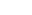has tangent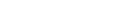then this can be expressed as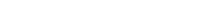which says that the covariant derivative
Covariant derivative
In mathematics, the covariant derivative is a way of specifying a derivative along tangent vectors of a manifold. Alternatively, the covariant derivative is a way of introducing and working with a connection on a manifold by means of a differential operator, to be contrasted with the approach given...

of the tangent in the direction of the tangent is zero. The above equation can be restated in terms of components of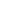: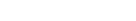where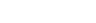and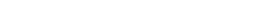The full geodesic equation is therefore: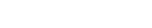where s is the proper time
Proper time
In relativity, proper time is the elapsed time between two events as measured by a clock that passes through both events. The proper time depends not only on the events but also on the motion of the clock between the events. An accelerated clock will measure a smaller elapsed time between two...

or distance and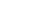is the Levi-Civita connection
Levi-Civita connection
In Riemannian geometry, the Levi-Civita connection is a specific connection on the tangent bundle of a manifold. More specifically, it is the torsion-free metric connection, i.e., the torsion-free connection on the tangent bundle preserving a given Riemannian metric.The fundamental theorem of...

.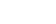,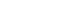,,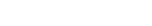,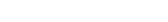,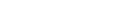The parameter s typically represents proper time
Proper time
In relativity, proper time is the elapsed time between two events as measured by a clock that passes through both events. The proper time depends not only on the events but also on the motion of the clock between the events. An accelerated clock will measure a smaller elapsed time between two...

for a timelike curve, or distance for a spacelike curve. This parameter cannot be chosen arbitrarily. Rather, it must be chosen so that the tangent vector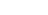has constant magnitude. This is referred to as an affine parametrization. Any two affine parameters are linearly related. That is, if r and s are affine parameters, then there exist constants a and b such that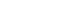.

## Geodesics as extremal curves

A geodesic between two events could also be described as the curve joining those two events which has the maximum possible length in time — for a timelike curve — or the minimum possible length in space — for a spacelike curve. The four-length of a curve in spacetime is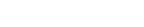Then, the Euler-Lagrange equation
Euler-Lagrange equation
In calculus of variations, the Euler–Lagrange equation, Euler's equation, or Lagrange's equation, is a differential equation whose solutions are the functions for which a given functional is stationary...

,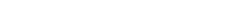becomes, after some calculation,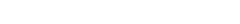The goal being to extremize the value of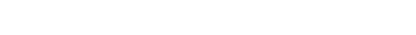where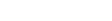such goal can be accomplished by calculating the Euler-Lagrange equation for f, which is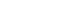.

Substituting the expression of f into the Euler-Lagrange equation (which extremizes the value of the integral l), gives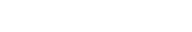Now calculate the derivatives: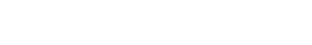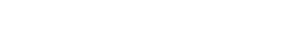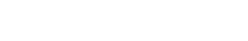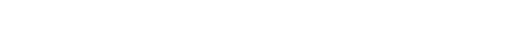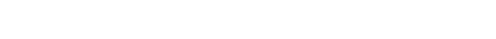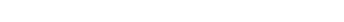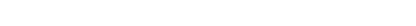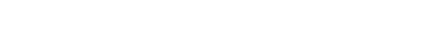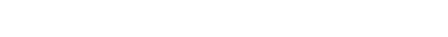This is just one step away from the geodesic equation.

If the parameter s is chosen to be affine, then the right side the above equation vanishes (because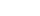is constant). Finally, we have the geodesic equation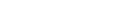## Geodesic incompleteness and singularities

The notion of geodesic incompleteness is used in the study of gravitational singularities.

## Approximate geodesic motion

True geodesic motion is an idealization where one assumes the existence of test particle
Test particle
In physical theories, a test particle is an idealized model of an object whose physical properties are assumed to be negligible except for the property being studied, which is considered to be insufficient to alter the behavior of the rest of the system...

s. Although in many cases real matter and energy can be approximated as test particles, situations arise where their appreciable mass (or equivalent thereof) can affect the background gravitational field in which they reside.

This creates problems when performing an exact theoretical description of a gravitational system (for example, in accurately describing the motion of two stars in a binary star system). This leads one to consider the problem of determining to what extent any situation approximates true geodesic motion. In qualitative terms, the problem is solved: the smaller the gravitational field produced by an object compared to the gravitational field it lives in (for example, the Earth's field is tiny in comparison with the Sun's), the closer this object's motion will be geodesic.

As Einstein's field equations
Einstein field equations
The Einstein field equations or Einstein's equations are a set of ten equations in Albert Einstein's general theory of relativity which describe the fundamental interaction of gravitation as a result of spacetime being curved by matter and energy...

determine the geometry of spacetime, it should be possible to determine the geodesics of the spacetime as well. For the case of dust
Dust (relativity)
In special and general relativity, dust is the name conventionally given to a configuration of matter which can be interpreted as small bodies which interact only gravitationally....

, the problem can be solved by using the Bianchi identities. Many attempts have been made to do the same for other matter distributions.